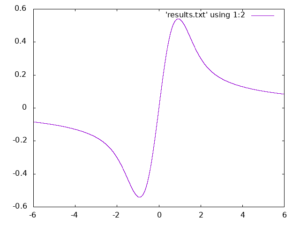## Installing and using the GNU Scientific Library (GSL) on the Windows Subsystem for Linux

January 23rd, 2019

I have been an advocate of the Windows Subsytem for Linux ever since it was released (See Bash on Windows: The scripting game just changed) since it allows me to use the best of Linux from my windows laptop.  I no longer dual boot on my personal machines and rarely need to use Linux VMs on them either thanks to this technology.  I still use full-blown Linux a lot of course but these days it tends to be only on servers and HPC systems.

I recently needed to compile and play with some code that was based on the GNU Scientific Library. Using the Ubuntu 18.04 version of the WSL this is very easy. Install the GSL with

```sudo apt-get install libgsl-dev
```

A simple code that evaluates Dawson’s integral over a range of x values is shown below. Call this dawson.cpp

```#include<iostream>
#include<vector>
#include<gsl/gsl_sf.h>

int main(){

double range = 6; // max/min values
int N = 100000; // Number of evaluations
double step = 2 * range / N;
std::vector<double> x(N);
std::vector<double> result(N);

for (int i=0;i<=N;i++){
x[i] = -range + i*step;
result[i] = gsl_sf_dawson(x[i]);
}

for (int i=0;i<=N;i++){
std::cout << x[i] << "," << result[i] << std::endl;
}

return 0;
}
```

Compile with

```g++ -std=c++11 dawson.cpp -o ./dawson -lgsl -lgslcblas -lm
```

Run it and generate some results

```./dawson > results.txt
```

If we plot results.txt we getThis code is also available on GitHub: https://github.com/mikecroucher/GSL_example